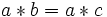# Cancellative element

(Redirected from Right cancellative element)

This article defines a property of elements or tuples of elements with respect to a binary operation

## Definition

An element$a$ in a magma$(S,*)$ (a set$S$ with binary operation$*$) is termed:

• left-cancellative if whenever$a * b = a * c$,$b = c$
• right-cancellative if whenever$b * a = c * a$,$b = c$
• cancellative if it is both left and right cancellative

A magma where every element is left-cancellative (resp. right-cancellative, cancellative) is termed a left-cancellative magma (resp., right-cancellative magma, cancellative magma).